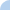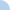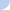Guess and Check factoring a trinomial!

For this quiz, you are given a trinomial or binomial. You need to select the answer which, when multiplied, will yield the thing that you started with!! For example, if you are given x²+5x+4, you would select
(x+4)(x+1), since when you multiply (x+4)(x+1) you end up getting x²+5x+4. You may take this quiz many times -- I will only get your best result!Quiz Log In